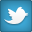###Author Topic: Average percentage calculation  (Read 2717 times)

####hem852

• Full Member
•• Join Date: Apr 2008
• Posts: 31
• Forum Citizenship: +0/-0##### Average percentage calculation
« on: 16 May 2008 09:57:18 am »
Hi

I have a cube which has a few measures that give straightforward values and one measure that gives a percentage

In the cube I have a calculated category titled

'Average per week for last 12 weeks'

and a Special category titled

'Last 12 weeks'

The 'Average per week for last 12 weeks' calculated category is causing me some problems. If for this category I use the calculation

Average("Cat number") ----- (Where Cat number is the category number for 'Last 12 weeks' Special category.)

The cube correctly calculates Average Values per week for the percentage measure but values for other measures are multiplied by 12.

If instead of using the Average function I use the following calculation for the same calculated category

Cat number / 12 ----- (Where Cat number is the category number for 'Last 12 weeks' Special category.)

The cube correctly calculates Average Values per week for all measures except the percentage which appears to get divided by 12.

Is there a way to get this cube to produce accurate average values for all measures?

Regards
John
John Daisley
Spirax Sarco Plc.
http://www.spiraxsarco.com